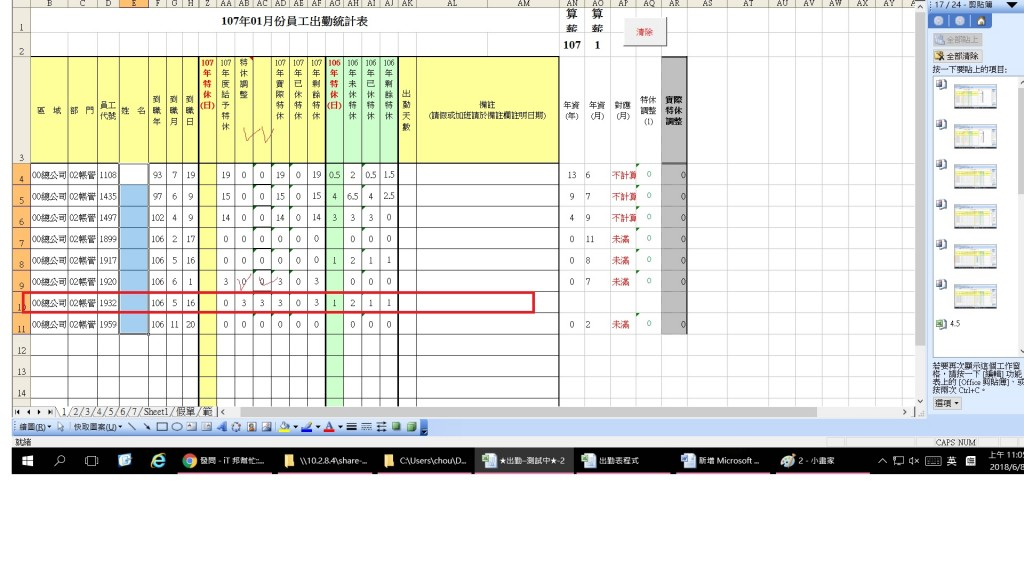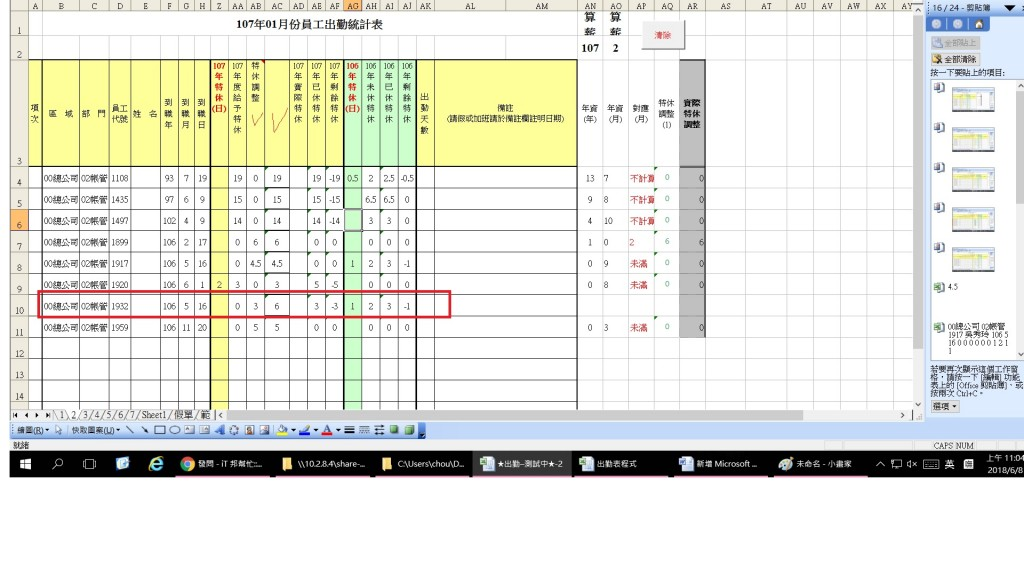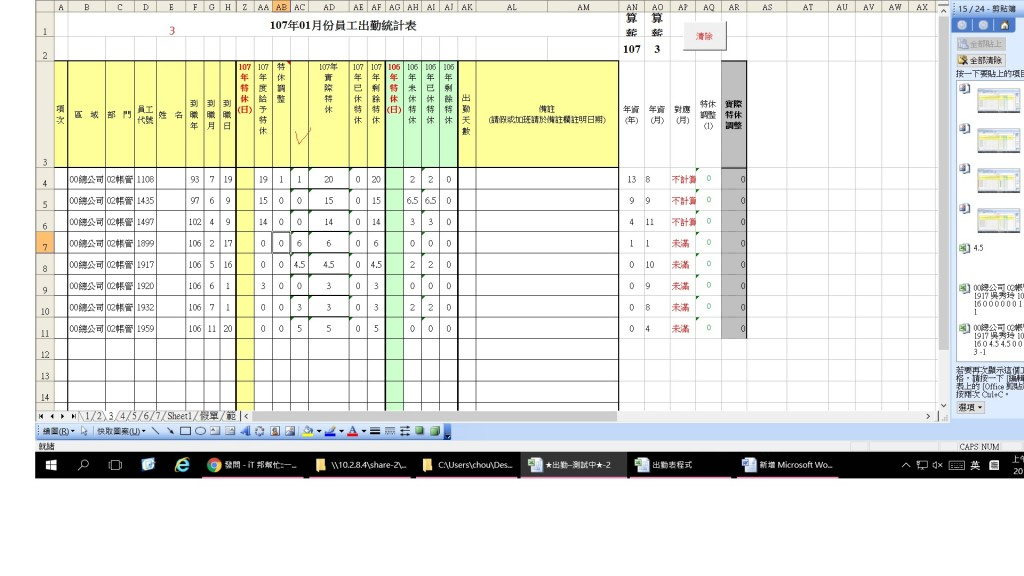#0

## EXCEL 公式累加

123swhung iT邦新手 5 級 ‧ 2018-06-08 11:49:11 檢舉
2003
swhung iT邦新手 5 級 ‧ 2018-06-08 12:56:08 檢舉

### 1 個回答

0

VLOOKUP的地方，他會去抓前面欄位的資料，但是因為前面沒有8000的資料而出錯

``````=IF(MID(CELL("filename",\$A\$1),FIND("]",CELL("filename",\$A\$1))+1,255)="1",AB11,AB11+VLOOKUP(D11,INDIRECT(LEFT(MID(CELL("filename",\$A\$1),FIND("]",CELL("filename",\$A\$1))+1,255),LEN(MID(CELL("filename",\$A\$1),FIND("]",CELL("filename",\$A\$1))+1,255))-0)-1&"!D:AD"),25,FALSE))+'1'!AA11
``````

``````=IF(MID(CELL("filename",\$A\$1),FIND("]",CELL("filename",\$A\$1))+1,255)="4",AB11,AB11+VLOOKUP(D11,INDIRECT(LEFT(MID(CELL("filename",\$A\$1),FIND("]",CELL("filename",\$A\$1))+1,255),LEN(MID(CELL("filename",\$A\$1),FIND("]",CELL("filename",\$A\$1))+1,255))-0)-1&"!D:AD"),25,FALSE))+'4'!AA11
``````